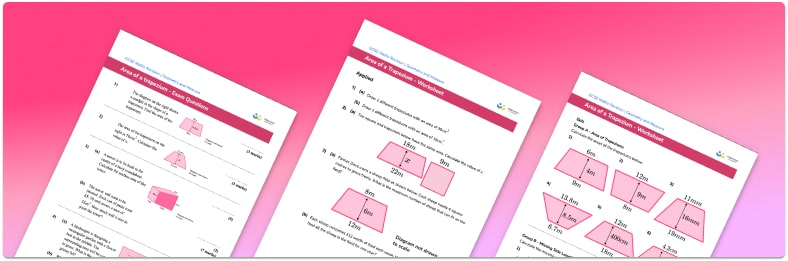# Area Of A Trapezium Worksheet• Section 1 of the area of trapezium worksheet contains 15 skills-based area of trapezium questions, in 3 groups to support differentiation
• Section 2 contains 4 applied area of trapezium questions with a mix of worded problems and deeper problem solving questions
• Section 3 contains 4 foundation and higher level GCSE exam style area of trapezium questions
• Answers and a mark scheme for all area of trapezium questions are provided
• Questions follow variation theory with plenty of opportunities for students to work independently at their own level
• All questions created by fully qualified expert secondary maths teachers
• Suitable for GCSE maths revision for AQA, OCR and Edexcel exam boards

• This field is for validation purposes and should be left unchanged.

You can unsubscribe at any time (each email we send will contain an easy way to unsubscribe). To find out more about how we use your data, see our privacy policy.

### Area of a trapezium at a glance

Area is the amount of space covered within the perimeter of a 2D shape. The area is measured in square units – such as m², cm² and mm². At GCSE level, students should be able to find the area of a number of different quadrilaterals, such as the area of a trapezoid or trapezium.

The formula for the area of a trapezium is based on the area of a rectangle. To calculate the area of a trapezoid, use the formula (½)(a+b)h, where h is the height of the trapezium and a and b are the lengths of the two parallel sides.

A right angled trapezium is one where the angle between each parallel side and the height is 90°. An alternative method to find the area of the trapezoid is to split it into a rectangle and a triangle, and then use the standard rectangle area formula and triangle area formula before adding the areas together.

Students are sometimes given the area of the trapezium and are then asked to use inverse operations to find a missing side length – for this, it helps if students are familiar with algebraic rearrangement.

In addition to using integers for the sides of the trapezoid, fractions and decimals are sometimes used. Students also need to be able to round their answer to a given number of decimal places and convert between different metric units – for example, if one side is given in centimetres and the other in millimetres.

Looking forward, students can then progress to additional area worksheets and other geometry worksheets, for example an angles in polygons worksheet or area and circumference of a circle worksheet.For more teaching and learning support on Geometry our GCSE maths lessons provide step by step support for all GCSE maths concepts.

## Do you have KS4 students who need more focused attention to succeed at GCSE?There will be students in your class who require individual attention to help them succeed in their maths GCSEs. In a class of 30, it’s not always easy to provide.

Help your students feel confident with exam-style questions and the strategies they’ll need to answer them correctly with our dedicated GCSE maths revision programme.

Lessons are selected to provide support where each student needs it most, and specially-trained GCSE maths tutors adapt the pitch and pace of each lesson. This ensures a personalised revision programme that raises grades and boosts confidence.

Find out more